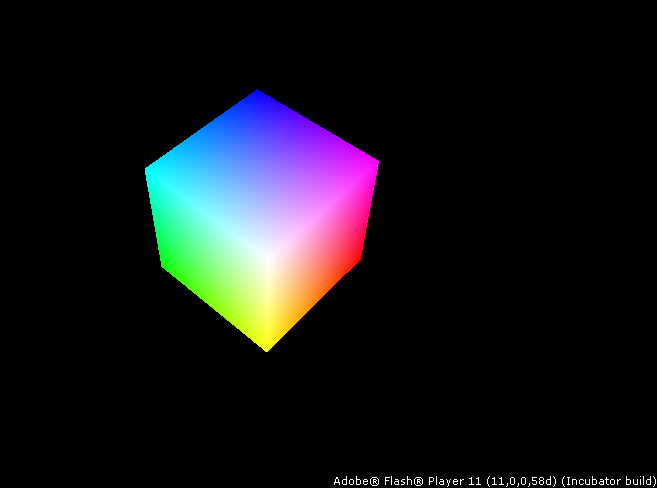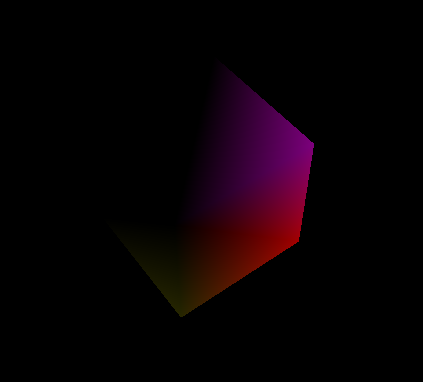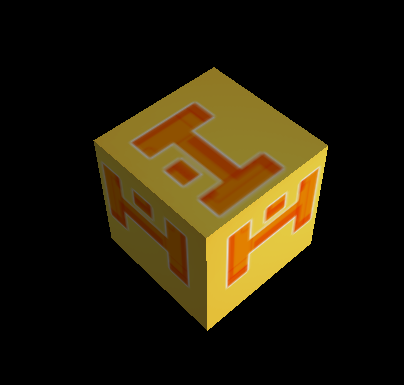# 安装

• 下载Flash Player 11(或更高版本) ，点这里下载 Adobe
• 安装 Haxe 2.08 Release
• 下载并解压molehill.zip Haxe 例子（含基本的3d类库：顶点，uv等)
• 当Haxe安装后，运行下面命令行安装第三方库 `format` :
`haxelib install format`

# 例子 0 - 立方体`new` 函数 (Haxe 类构造函数), 我们装配一个新的3D场景(3D stage)用于3D渲染 . 代码如下 :

```var s : flash.display.Stage3D;
//...
stage = flash.Lib.current.stage;
s = stage.stage3Ds;
s.requestContext3D();```

```var c : flash.display3D.Context3D;
var pol : Polygon;
var camera : Camera;
// ...
c = s.context3D;
c.enableErrorChecking = true;
c.configureBackBuffer( stage.stageWidth, stage.stageHeight, 0, true );

camera = new Camera();

pol = new Cube();
pol.alloc(c);
}```

`update`函数内，我们将开始装配一些3D环境（context 3D）需要的内容。

```c.clear(0, 0, 0, 1);
c.setDepthTest( true, flash.display3D.Context3DCompareMode.LESS_EQUAL );
c.setCulling(flash.display3D.Context3DTriangleFace.BACK);```

```if( keys[K.UP] )
camera.moveAxis(0,-0.1);
if( keys[K.DOWN] )
camera.moveAxis(0,0.1);
if( keys[K.LEFT] )
camera.moveAxis(-0.1,0);
if( keys[K.RIGHT] )
camera.moveAxis(0.1, 0);
if( keys )
camera.zoom /= 1.05;
if( keys )
camera.zoom *= 1.05;
camera.update();```

```var project = camera.m.toMatrix();
var mpos = new flash.geom.Matrix3D();
mpos.appendRotation(t * 10, flash.geom.Vector3D.Z_AXIS);```

```shader.init(
{ mpos : mpos, mproj : project },
{}
);
c.drawTriangles(pol.ibuf);
c.present();```

```class Shader extends format.hxsl.Shader {
static var SRC = {
var input : {
pos : Float3,
};
var color : Float3;
function vertex( mpos : M44, mproj : M44 ) {
out = pos.xyzw * mpos * mproj;
color = pos;
}
function fragment() {
out = color.xyzw;
}
}
}```

`@:shader` section contains Haxe Metadata which is compiled at the same time you're compiling your project by using Haxe .

# 例子 1 - 灯光 Lighting

`pol.addNormals();`

This will calculate a per-vertex normal. Since the cube points are shared by triangles faces, you'll get very smooth normals.

```var light = new flash.geom.Vector3D(
Math.cos(t * 10) * 1,
Math.sin(t * 5) * 2,
Math.sin(t) * Math.cos(t) * 2
);
light.normalize();
{ mpos : mpos, mproj : project, light : light },
{}
);```

```var tnorm = (norm * mpos).normalize();
var lpow = light.dot(tnorm).max(0);
color = pos * lpow;````pol.unindex();`

# 例子 2 - 材质贴图 Texturing

`pol.addTCoords();`

```@:bitmap("hxlogo.png")
class HaxeLogo extends flash.display.BitmapData {
}
// ...
var logo = new HaxeLogo();
texture = c.createTexture(logo.width, logo.height, flash.display3D.Context3DTextureFormat.BGRA, false);
```

```shader.init(
{ mpos : mpos, mproj : project, light : light },
{ tex : texture }
);```

```var input : {
pos : Float3,
norm : Float3,
uv : Float2,
};
var tuv : Float2;
var lpow : Float;
function vertex( mpos : M44, mproj : M44, light : Float3 ) {
out = pos.xyzw * mpos * mproj;
var tnorm = (norm * mpos).normalize();
lpow = light.dot(tnorm).max(0);
tuv = uv;
}
function fragment( tex : Texture ) {
out = tex.get(tuv) * (lpow * 0.8 + 0.2);
}```version #12118, modified 2011-12-31 17:00:26 by haha123_0